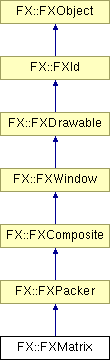Main Page   Class Hierarchy   Alphabetical List   Compound List   File List   Compound Members# FX::FXMatrix Class Reference

The Matrix layout manager automatically arranges its child windows in rows and columns. More...

`#include <FXMatrix.h>`

Inheritance diagram for FX::FXMatrix:List of all members.

## Public Member Functions

FXMatrix (FXComposite *p, FXint n=1, FXuint opts=MATRIX_BY_ROWS, FXint x=0, FXint y=0, FXint w=0, FXint h=0, FXint pl=DEFAULT_SPACING, FXint pr=DEFAULT_SPACING, FXint pt=DEFAULT_SPACING, FXint pb=DEFAULT_SPACING, FXint hs=DEFAULT_SPACING, FXint vs=DEFAULT_SPACING)
virtual void layout ()
virtual FXint getDefaultWidth ()
virtual FXint getDefaultHeight ()
FXWindowchildAtRowCol (FXint r, FXint c) const
FXint rowOfChild (const FXWindow *child) const
FXint colOfChild (const FXWindow *child) const
void setMatrixStyle (FXuint ph)
FXuint getMatrixStyle () const
void setNumRows (FXint nr)
FXint getNumRows () const
void setNumColumns (FXint nc)
FXint getNumColumns () const

## Detailed Description

The Matrix layout manager automatically arranges its child windows in rows and columns.

If the matrix style is MATRIX_BY_ROWS, then the matrix will have the given number of rows and the number of columns grows as more child windows are added; if the matrix style is MATRIX_BY_COLUMNS, then the number of columns is fixed and the number of rows grows as more children are added. If all children in a row (column) have the LAYOUT_FILL_ROW (LAYOUT_FILL_COLUMN) hint set, then the row (column) will be stretchable as the matrix layout manager itself is resized. If more than one row (column) is stretchable, the space is apportioned to each stretchable row (column) proportionally. Within each cell of the matrix, all other layout hints are observed. For example, a child having LAYOUT_CENTER_Y and LAYOUT_FILL_X hints will be centered in the Y-direction, while being stretched in the X-direction. Empty cells can be obtained by simply placing a borderless FXFrame widget as a space-holder.

## Constructor & Destructor Documentation

 FX::FXMatrix::FXMatrix ( FXComposite * p, FXint n = `1`, FXuint opts = `MATRIX_BY_ROWS`, FXint x = `0`, FXint y = `0`, FXint w = `0`, FXint h = `0`, FXint pl = `DEFAULT_SPACING`, FXint pr = `DEFAULT_SPACING`, FXint pt = `DEFAULT_SPACING`, FXint pb = `DEFAULT_SPACING`, FXint hs = `DEFAULT_SPACING`, FXint vs = `DEFAULT_SPACING` )
 Construct a matrix layout manager with n rows or columns.

## Member Function Documentation

 virtual void FX::FXMatrix::layout ( ) ` [virtual]`
 Perform layout. Reimplemented from FX::FXPacker.

 virtual FXint FX::FXMatrix::getDefaultWidth ( ) ` [virtual]`
 Return default width. Reimplemented from FX::FXPacker.

 virtual FXint FX::FXMatrix::getDefaultHeight ( ) ` [virtual]`
 Return default height. Reimplemented from FX::FXPacker.

 FXWindow* FX::FXMatrix::childAtRowCol ( FXint r, FXint c ) const
 Obtain the child placed at a certain row and column.

 FXint FX::FXMatrix::rowOfChild ( const FXWindow * child ) const
 Return the row in which the given child is placed.

 FXint FX::FXMatrix::colOfChild ( const FXWindow * child ) const
 Return the column in which the given child is placed.

 void FX::FXMatrix::setMatrixStyle ( FXuint ph )
 Change the matrix style.

 FXuint FX::FXMatrix::getMatrixStyle ( ) const
 Return the current matrix style.

 void FX::FXMatrix::setNumRows ( FXint nr )
 Change the number of rows.

 FXint FX::FXMatrix::getNumRows ( ) const
 Return the number of rows.

 void FX::FXMatrix::setNumColumns ( FXint nc )
 Change the number of columns.

 FXint FX::FXMatrix::getNumColumns ( ) const
 Return the number of columns.Copyright © 1997-2005 Jeroen van der Zijp